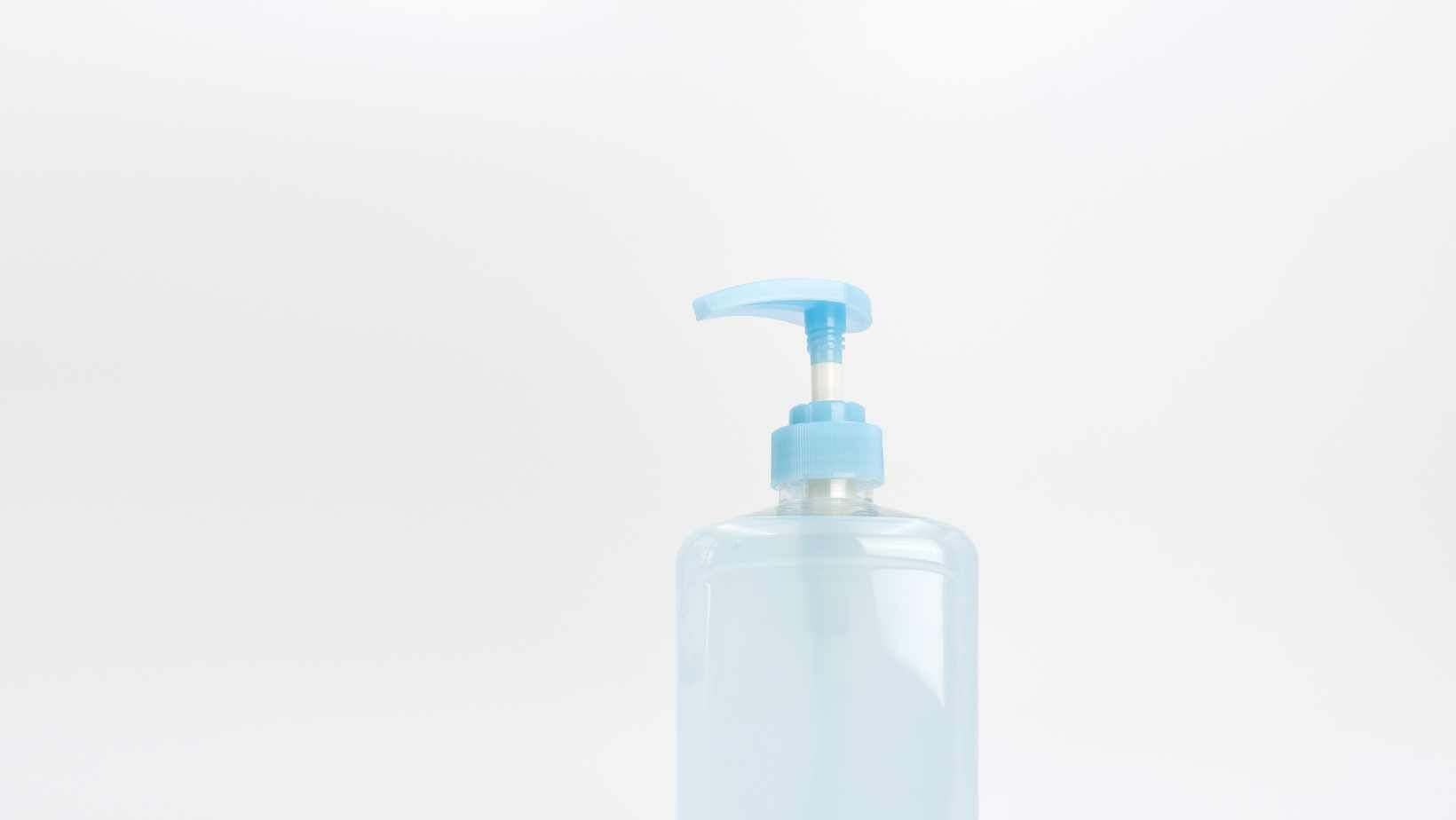# How Many Ounces in a 750 ml Bottle? The Ultimate DiscoveryIf you’ve ever found yourself wondering how many ounces are in a 750 ml bottle, you’re not alone. The conversion between milliliters and ounces can be confusing, especially when dealing with common bottle sizes like the 750 ml. So, let’s get straight to the answer: there are approximately 25.36 ounces in a 750 ml bottle.

To put it into perspective, a standard wine bottle is typically 750 ml in volume, which equates to around 25.36 fluid ounces. This measurement is widely used for wines and other spirits around the world. Knowing this conversion can come in handy when comparing drink sizes or trying to understand serving recommendations.

## How Many Ounces in a 750 ML

### Converting Fluid Ounces to Milliliters

When it comes to understanding the measurement of liquids, one common question that arises is “how many ounces are in a 750 ml?” To find the answer, we need to explore the conversion rates between fluid ounces and milliliters.

In the United States, fluid ounces (fl oz) are commonly used as a unit of volume measurement. On the other hand, milliliters (ml) are widely used in many other countries around the world. To convert between these two measurements, we must keep in mind that 1 fluid ounce is approximately equal to 29.5735 milliliters.

To calculate how many ounces there are in a 750 ml bottle, we can use this conversion rate. By dividing 750 ml by approximately 29.5735 ml/fl oz, we find that there are about 25.36 fluid ounces in a standard 750 ml bottle.

### Understanding the Standard Measurement: Fluid Ounces

Fluid ounces represent volume and are commonly used for measuring liquid ingredients in recipes or serving sizes for beverages. Whether you’re pouring yourself a glass of water or following a recipe’s instructions, understanding how much liquid is equivalent to an ounce can be helpful.

It’s important to note that fluid ounces differ from weight ounces (oz). Weight ounces measure mass rather than volume and are typically used for solid objects like fruits or vegetables on food scales.

### Exploring the Conversion Rates: Fluid Ounces to Milliliters

The conversion from fluid ounces to milliliters is based on their respective volumes. As mentioned earlier, one fluid ounce is approximately equal to 29.5735 milliliters.

To convert from fluid ounces to milliliters, you can use this simple formula:

milliliters = fluid_ounces * 29.5735

For example, if you have 10 fluid ounces and want to know the equivalent in milliliters, you can multiply 10 by 29.5735 to get approximately 295.74 milliliters.

Here’s a useful table that showcases the conversion rates for common measurements:

 Fluid Ounces Milliliters 1 29.57 5 147.87 10 295.74 16 473.18 32 946.35

Remember, these values are approximate since the conversion rate is based on a rounded number.

In conclusion, when it comes to understanding how many ounces are in a standard bottle of 750 ml, we find that there are approximately 25.36 fluid ounces. Understanding the conversion rates between fluid ounces and milliliters allows us to accurately measure and convert liquid volumes according to our needs.

## Decoding The Metric System: Milliliters

When it comes to understanding the metric system, milliliters play a crucial role in measuring liquids. If you’ve ever wondered how many ounces are in a 750 ml bottle, let’s dive into the conversion and shed some light on this common query.

To convert milliliters to ounces, we need to consider that 1 fluid ounce is equivalent to approximately 29.57 milliliters. So, when we have a 750 ml bottle, what does that translate to in terms of ounces?

To find out, we can use a simple calculation:

750 ml * (1 fl oz / 29.57 ml) = X fl oz

By multiplying the volume in milliliters by the conversion factor (1 fluid ounce divided by 29.57 milliliters), we can determine the equivalent value in fluid ounces.

Calculating this equation gives us:

750 * (1/29.57) ≈ 25.36 fl oz

Therefore, there are approximately 25.36 fluid ounces in a standard 750 ml bottle.

It’s important to note that this conversion is an approximation since fluid ounces and milliliters are not exact equivalents. However, for most practical purposes, this estimation will suffice.

In conclusion:

• A standard 750 ml bottle is roughly equivalent to about 25.36 fluid ounces.
• To convert milliliters to fluid ounces, multiply the volume by the conversion factor of approximately (1/29.57).
• Remember that these conversions provide approximate values due to slight variations between fluid ounces and milliliters.

I hope this clarifies any confusion surrounding how many ounces are found in a standard 750 ml bottle!

Amanda is the proud owner and head cook of her very own restaurant. She loves nothing more than experimenting with new recipes in the kitchen, and her food is always a big hit with customers. Amanda takes great pride in her work, and she always puts her heart into everything she does. She's a hard-working woman who has made it on her own, and she's an inspiration to all who know her.